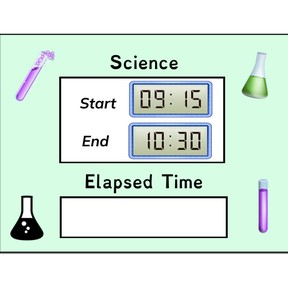Time difference: Digital clocks with quarter hours

# Time difference: Digital clocks with quarter hours

I can determine time differences between digital clocks with quarter hours.

No account needed.8,000 schools use Gynzy92,000 teachers use Gynzy1,600,000 students use Gynzy

## General

In this lesson, students will practice calculating the elapsed time between two digital clocks with quarter hours. Students will be given the skills to find the time that has passed between two events. They will learn to utilize various strategies, like number lines and t-charts to calculate elapsed time with quarter hours. Then, students will complete several activities in which they practice calculating elapsed time.

## Standards

CCSS.Math.Content.3.MD.A.1

## Learning Objective

Students will be able to properly tell what the difference is between two digital clocks with whole, half, and quarter hours.

## Introduction

Students will review what elapsed time is and discuss their answer to a word problem with their classmates, noting the steps they took to reach their answer.

## Instruction

The lesson will begin with students adjusting two clocks to reflect when they wake up and go to sleep every day. Students will discuss how much time they think elapses between the two. Next, students will be guided into completing a KWL chart about calculating elapsed time with digital clocks and quarter hours.

Explain how to utilize number lines and t-charts to solve elapsed word problems through guided examples and videos. Then, have students practice applying the knowledge they learned on their own through several activities such as helping Mrs. Miller determine the time elapsed between each of her classes.

## Quiz

Students respond to ten questions in which they practice calculating elapsed time.

## Closing

Students will complete an activity in which they imagine bees are busy retrieving honey from honeycombs. They will solve for each elapsed time by looking at the provided start and end times.

### The online teaching platform for interactive whiteboards and displays in schools

• Save time building lessons

• Manage the classroom more efficiently

• Increase student engagement